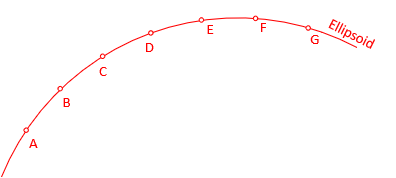### 3. A matter of Scale

#### a. Distance Distortion

Distances are distorted when projected from the ellipsoid to a developable surface. The distortion can be stated as a ratio or a scale.

• A ratio is a unit distortion per a specific distance. A ratio of 1:5,000 or 1/5,000 means a 5,000 ft distance will be distorted by ± 1 ft, a 10,000 ft by ±2 ft, etc.  A 1/10,000 distortion is less than 1/5,000.
• A scale is a ration converted to a multiplier. Since the distortion can be ±, its scale is a range. 1/10,000 as a scale is a range between (1-1/10,000) and (1+1/10,000) which is 0.9999 to 1.0001.

Scale is systematic and can be compensated mathematically. However, for many applications, grid and geodetic distances can be considered the same below some maximum scale. This can reduce computations substantially.

Surface fit and area extent affect how distance distortion behaves.

#### b. Surface Fit

A developable surface can be either tangent or secant to the ellipsoid.

• A tangent fit touches the ellipsoid at a single point (plane) or along a line (cylinder and cone).
• A secant fit cuts through the ellipsoid along an ellipse (plane) or two parallel lines (cylinder and cone).

Figure D-7 shows an ellipsoid section with evenly spaced points.Figure D-7 Points on the Ellipsoid

Except where it intersects the ellipsoid, a tangent surface is everywhere outside the ellipsoid, Figure D-8.Figure D-8 Tangent Surface Fit

Points are projected to the surface, displacing them outward from the tangent location. The further away, the greater the displacement. Distances increase accordingly: D'E' < E'F' < F'G'. Scale at the tangent location is exactly 1 and gets larger further away. Scale is never less than 1 on a tangent surface.

A secant surface is part inside and part outside the ellipsoid, Figure D-9.Figure D-9 Secant Surface Fit

Ellipsoid points projected inward to the surface shorten the distances in between; Scale<1.
Ellipsoid points projected outward to the surface lengthen the distances in between; Scale>1.
Scale is 1 at the intersection where there is no displacement.

Because a tangent surface's scale is always equal to or greater than 1, its use is generally limited to a smaller area. A secant surface can cover a greater area since its scale varies between less than and greater than 1. Most commonly used regional grid systems are based on secant projections.